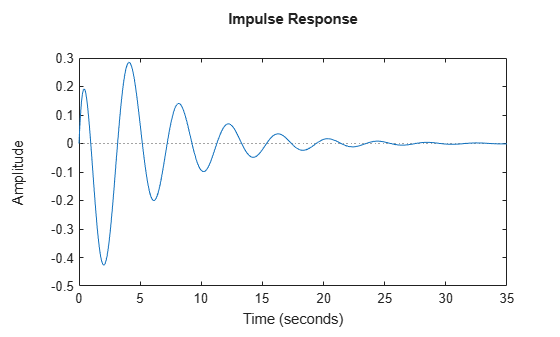# lsiminfo

Compute linear response characteristics

## Syntax

``S = lsiminfo(y,t)``
``S = lsiminfo(y,t,yfinal)``
``S = lsiminfo(y,t,yfinal,yinit)``
``S = lsiminfo(___,'SettlingTimeThreshold',ST)``

## Description

`lsiminfo` lets you compute linear response characteristics from an array of response data `[y,t]`. For a linear response y(t), `lsiminfo` computes characteristics relative to yinit and yfinal, where yinit is the initial offset, that is, the value before the input is applied, and yfinal is the steady-state value of the response.

`lsiminfo` uses yinit = 0 and yfinal = last sample value of y(t) unless you explicitly specify these values.

The function returns the characteristics in a structure containing the fields:

• `TransientTime` — The first time T such that the error |y(t) – yfinal| ≤ SettlingTimeThreshold × emax for tT, where emax is the maximum error |y(t) – yfinal| for t ≥ 0.

By default, SettlingTimeThreshold = 0.02 (2% of the peak error). Transient time measures how quickly the transient dynamics die off.

• `SettlingTime` — The first time T such that |y(t) – yfinal| ≤ SettlingTimeThreshold × |yfinalyinit| for tT.

By default, settling time measures the time it takes for the error to stay below 2% of |yfinalyinit|.

• `Min` — Minimum value of y(t).

• `MinTime` — Time the response takes to reach the minimum value.

• `Max` — Maximum value of y(t).

• `MaxTime` — Time the response takes to reach the maximum value.

````S = lsiminfo(y,t)` computes linear response characteristics from an array of response data `y` and corresponding time vector `t`. This syntax uses yinit = 0 and the last value in `y` (or the last value in each channel's corresponding response data) as yfinal to compute characteristics that depend on these values.For SISO system responses, `y` is a vector with the same number of entries as `t`. For MIMO response data, `y` is an array containing the responses of each I/O channel.```

example

````S = lsiminfo(y,t,yfinal)` computes linear response characteristics relative to the steady-state value `yfinal`. This syntax is useful when you know that the expected steady-state system response differs from the last value in y for reasons such as measurement noise. This syntax uses yinit = 0.For SISO responses, `t` and `y` are vectors with the same length `NS`. For systems with `NY` outputs, you can specify `y` as an `NS`-by-`NY` array and `yfinal` as an `NY`-by-`1` array. `lsiminfo` then returns a `NY`-by-`1` structure array `S` of response characteristics corresponding to each output channel.```
````S = lsiminfo(y,t,yfinal,yinit)` computes response characteristics relative to the response initial value `yinit`. This syntax is useful when your `y` data has an initial offset, that is, `y` is nonzero before the input is applied.For SISO responses, `t` and `y` are vectors with the same length `NS`. For systems with `NY` outputs, you can specify `y` as an `NS`-by-`NY` array and `yfinal` and `yinit` as an `NY`-by-`1` arrays. `lsiminfo` then returns a `NY`-by-`1` structure array `S` of response characteristics corresponding to each output channel.```
````S = lsiminfo(___,'SettlingTimeThreshold',ST)` lets you specify the threshold `ST` used in definition of settling and transient times. The default value is `ST = 0.02` (2%). You can use this syntax with any of the previous input-argument combinations.```

## Examples

collapse all

Create the following continuous-time transfer function:

`$H\left(s\right)=\frac{s-1}{{s}^{3}+2{s}^{2}+3s+4}$`

`sys = tf([1 -1],[1 2 3 4]);`

Calculate the impulse response.

`[y,t] = impulse(sys);`

`impulse` returns the output response `y` and the time vector `t` used for simulation.

Compute the response characteristics using a final response value of `0`.

`s = lsiminfo(y,t,0)`
```s = struct with fields: TransientTime: 22.8700 SettlingTime: NaN Min: -0.4268 MinTime: 2.0088 Max: 0.2847 MaxTime: 4.0733 ```

You can plot the impulse response and verify these response characteristics. For example, the time at which the minimum response value (`MinTime`) is reached is approximately 2 seconds.

`impulse(sys)`## Input Arguments

collapse all

Response data, specified as one of the following:

• For SISO response data, a vector of length `Ns`, where `Ns` is the number of samples in the response data.

• For MIMO response data, an `Ns`-by-`Ny` array, where `Ny` is the number of system outputs.

Time vector corresponding to the response data in `y`, specified as a vector of length `Ns`.

Response steady-state value, specified as a scalar or an array.

• For SISO response data, specify a scalar value.

• For MIMO response data, specify an `Ny`-by-`1` array, where each entry provides the steady-state response value for the corresponding system channel.

If you do not provide `yfinal`, then `lsiminfo` uses the last value in the corresponding channel of `y` as the steady-state response value.

Value of `y` before the input is applied, specified as a scalar or an array.

• For SISO response data, specify a scalar value.

• For MIMO response data, specify an `Ny`-by-`1` array, where each entry provides the response initial value for the corresponding system channel.

If you do not provide `yinit`, then `lsiminfo` uses zero as the response initial value.

Threshold for defining settling and transient times, specified as a scalar value between 0 and 1. To change the default settling and transient time definitions (see Description), set `ST` to a different value. For instance, to measure when the error falls below 5%, set `ST` to 0.05.

## Output Arguments

collapse all

Linear response characteristics, returned as a structure containing the fields:

• `TransientTime`

• `SettlingTime`

• `Min`

• `MinTime`

• `Max`

• `MaxTime`

For more information on how `lsiminfo` defines these characteristics, see Description.

For MIMO models or responses data, `S` is a structure array in which each entry contains the step-response characteristics of the corresponding I/O channel. For instance, if you provide a 3-input, 3-output model or array of response data, then `S(2,3)` contains the characteristics of the response from the third input to the second output.

## Version History

Introduced in R2006a

expand all